Courses

# Number Systems - More Concepts Quant Notes | EduRev

## Quantitative Aptitude for SSC CGL

Created by: Wizius Careers

## Banking Exams : Number Systems - More Concepts Quant Notes | EduRev

The document Number Systems - More Concepts Quant Notes | EduRev is a part of the Banking Exams Course Quantitative Aptitude for SSC CGL.
All you need of Banking Exams at this link: Banking Exams

CYCLICITY
At times there are questions that require the students to find the units digit in case of the numbers occurring in powers. If anyone asks you to find the unit digit of 33, you will easily calculate it also you can calculate for 3but if any one ask you the unit digit of 17399, it will be hard to calculate easily.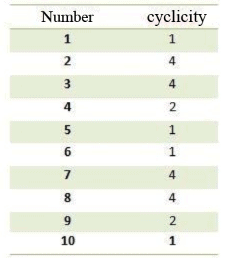But it’s very simple if we understand that the units digit of a product is determined by whatever is the digit at the units place irrespective of the number of digits.
Example: 5 × 5 ends in 5 & 625 × 625 also ends in 5.
Now let’s examine the pattern that a number generates when it occurs in powers of itself.
See the last digit of different numbers.

Table: Showing the Unit Digit of a Number for Different Exponents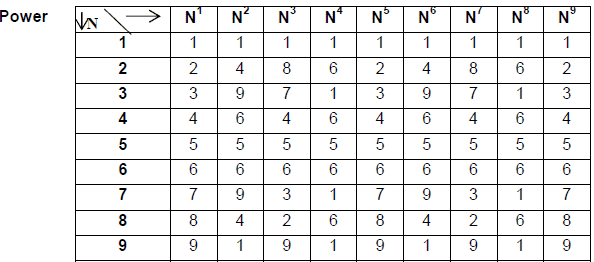From the above table we can conclude that the unit digit of a number repeats after
an interval of 1, 2 or 4. Precisely we can say that the universal cyclicity of all the
numbers is 4. i.e. after 4 all the numbers start repeating their unit digits.
Therefore, to calculate the unit digit for any exponent of a given number we have

Step 1: Divide the exponent of the given number by 4 and calculate the remainder.

Step 2: The unit digit of the number is same as the unit digit of the number raise to the power of calculated remainder.

Step 3: If the remainder is zero, then the unit digit will be same as the unit digit of N4.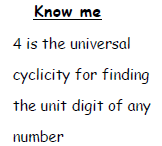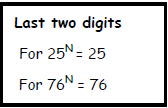LET US CONSIDER AN EXAMPLE
Example 1. Find the last digit of (173)99.
Solution. We notice that the exponent is 99. On dividing, 99 by 4 we get 24 as the quotient & 3 as the remainder.
Now these 24 pairs of 4 each do not affect the no. at the units place So, (173)99 ≈ (173)3. Now, the number at the units place is 33 = 27.

FACTORS
A factor is a number that divides another number completely. Example - Factors of 24 are: 1, 2, 3, 4, 6, 8, 12, 24.

Number of Factors
If we have a number, N = pa × qb × rc
Where p, q, and r are prime numbers and a, b, and c are the no. of times each
prime number occurs , then the number of factors of n is found by (a + 1) (b +1)(c + 1).
Example: Find the number of factors of 24 × 32.
Number of factors = (4 + 1) (2 + 1) = 5(3) = 15

Funda: All the perfect squares have odd number of factors and other number have even number of factors.

Number of Ways of Expressing a Given Number as a Product of Two Factors:

• When a number is having even number of factors then it can be written as a product of two numbers inways.
• But if a number have odd number of factors then it can be written as a product of two different numbers in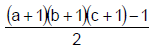ways and can be written as a product of two numbers (different or similar) in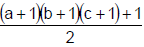ways.

Examples:
(i) 148 can be expressed as a product of two factors in 6/2 or 3 ways.
{Because (p + 1) (q + 1) (r + 1) in the case of 148 is equal to 6}.
(ii) 144 (24.32) can be written as a product of two different numbers in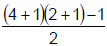i.e. 7 ways.

Sum of the factors of a number:
If a number N is written in the form of N = ap.bq.cr ,where a, b & c are prime numbers and p, q & r are positive integers, then the sum of all the factors of the number are given by the formula:
Sum of factors =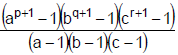FACTORIAL
Factorial is defined for any positive integer. It is denoted by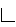or !. Thus “Factorial n” is written as n!
or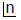n! is defined as the product of all the integers from 1 to n.
Thus n! = 1.2.3. …. n. (n! = n(n – 1)!)

Finding the Highest power of the number dividing a Factorial

Example 2. Find the largest power of 3 that can divide 95! without leaving any remainder.
Solution. First look at the detailed explanation and then look at a simpler method for solving the problem.
When we write 95! in its full form, we have 95 × 94 × 93 ….. × 3 × 2 × 1. When we divide 95! by a power of 3, we have these 95 numbers in the numerator. The denominator will have all 3’s. The 95 numbers in the numerator have 31 multiples of 3 which are 3, 6, 9….90, 93. Corresponding to each of these multiplies we can have a 3 in the denominator which will divide the numerator completely without leaving any remainder, i.e. 331 can definitely divide 95!.

Further every multiple of 9, i.e. 9, 18, 27, etc. after canceling out a 3 above, will still have one more 3 left. Hence for every multiple of 9 in the numerator, we have an additional 3 in the denominator. There are 10 multiples of 9 in 95 i.e. 9, 18….81, 90. So we can take 10 more 3’s in the denominator.

Similarly, for every multiple of 33 we can take an additional 3 in the denominator.
Since there are 3 multiples of 27 in 91 (they are 27, 54 and 81), we can have three more 3’s in the denominator.

Next, corresponding to every multiple of 34 i.e. 81 we can have one more 3 in the denominator. Since there is one multiple of 81 in 95, we can have one additional 3 in the denominator.

Hence the total number of 3’s we can have in the denominator is 31 + 10 + 3 + 1, i.e., 45. So 345 is the largest power of 3 that can divide 95! without leaving any remainder.

The same can be done in the simpler manner also which is explained below.
Divide 95 by 3 you get a quotient of 31. Divide this 31 by 3 we get a quotient of 10. Divide this 10 by 3 we get a quotient of 3. Divide this quotient of 3 once again by 3 we get a quotient of 1. Since we cannot divide the quotient any more by 3 we stop here. Add all the quotients, i.e. 31 + 10 + 3 + 1 which gives 45 which is the highest power of 3.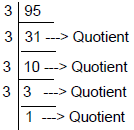Add all the quotients 31 + 10 + 3 + 1, which give 45.

{Note that this type of a division where the quotient of one step is taken as the dividend in the subsequent step is called “Successive Division”. In general, in successive division, the divisor need not be the same (as it is here). Here, the number 95 is being successively divided by 3}.

Note: This method is applicable only if the number whose largest power is to be found out is a prime number.

If the number is not a prime number, then we have to write the number as the product of relative primes, find the largest power of each of the factors separately first. Then the smallest, among the largest powers of all these relative factors of the given number will give the largest power required.

Example 3. Find the largest power of 12 that can divide 200!
Solution. Here we cannot apply Successive Division method because 12 is not a prime number. Resolve 12 into a set of prime factors. We know that 12 can be written as 3 × 4. So, we will find out the largest power of 3 that can divide 200! and the largest power of 4 that can divide 200! and take the LOWER of the two as the largest power of 12 that can divide 200!.
To find out the highest power of 4, since 4 itself is not a prime number, we cannot directly apply the successive division method. We first have to find out the highest power of 2 that can divide 200!. Since two 2’s taken together will give us a 4, half the power of 2 will give the highest power of 4 that can divide 200!. We find that 197 is the largest power of 2 that can divide 200!. Half this figure-98-will be the largest power of 4 that can divide 200!.

Since the largest power of 3 and 4 that can divide 200! are 97 an 98 respectively, the smaller of the two, i.e., 97 will be the largest power of 12 that can divide 200! without leaving any remainder.

Example 4. What is the last digit of 234 × 334 × 434
Solution. Given = (24)34
Last digit of 4n is 6, if n is even. ⇒ Answer 6

Example 5. What is the right most non zero digit of (270)270
Solution. The required answer is the last digit of 7270.
Last digit of 7 powers repeat after every 4.
So, the last digit of 7270 is the last digit of 72 = 9.

Example 6. How many factors do 1296 have?
Solution. 1296 = 4 × 324
= 4 × 4 × 81
= 24 × 34
Number of factors = (4 + 1) (4 + 1) = 25.

Example 7. If x is the sum of all the factors of 3128 and y is the no of factors of x and z is the number of ways of writing ‘y’ as a product of two numbers, then z = ?
Solution. 3128 = 4 × 782
= 4 × 2 × 391
= 23 × 17 × 23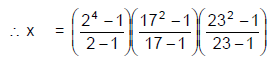= 15 × (17 + 1) (23 + 1)
= 3 × 5 × 9 × 2 × 8 × 3
= 24 × 34 × 5
∴ y = (4 + 1) (4 + 1) (1 + 1)
= 2 × 52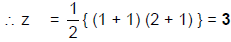Example 8. How many co-factors are there for 240, which are less than 240?
Solution.
240 = 16 × 15
= 24 × 3 × 5
Number of co primes to N, which are less than N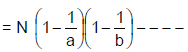if N = ab × bq × - - - - (a, b, - - - - are Prime no.s)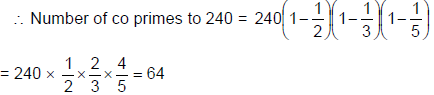Example 9. What is the sum of all the co primes to 748? Which are less than N?
Solution. 748 = 4 × 187
= 22 × 11 × 17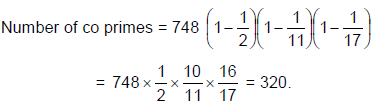Sum of all the co primes to N. which are less than N is N/2 (number of co primes to N, which are less
than N.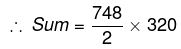= 119680

Example 10. In how many ways 5544 can be written as a product of 2 co primes?
Solution. If N = ap × bq × - - - -, where a, b, - - - - are prime numbers
N can be written as a product of two co primes in 2n-1 ways, where n is the number of prime factors to
N.
∴ 5544 = 11 × 504
= 11 × 9 × 56
= 11 × 9 × 8 × 7
= 23 × 32 × 7 × 11
Ans. = 24-1 = 23 = 8. (Because, 2, 3, 7 & 11 are four different prime factors).

Multiplying the last two digit of the exponents in ten seconds

Follow this trick and shortcut method to multiply the numbers in your head. This can be a huge advantage for those preparing for CAT exam or any other MBA entrance exams.
The tricks used in this video is simple and straight. You might not need a paper and pen to solve this type of question after learning the shortcuts.
The last digit of a number in form: an
The simple trick followed to know the last digit is to remember the cyclicity of the last digit of the number given. Every digit from 1 to 9 follow a pattern when exponential power is applied. Here is the table that will be handy in solving these type of questions.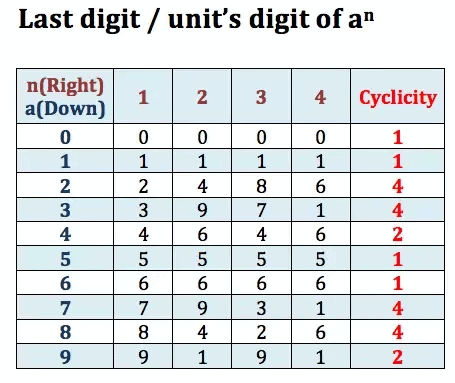Offer running on EduRev: Apply code STAYHOME200 to get INR 200 off on our premium plan EduRev Infinity!

## Quantitative Aptitude for SSC CGL

55 videos|52 docs|72 tests

,

,

,

,

,

,

,

,

,

,

,

,

,

,

,

,

,

,

,

,

,

;